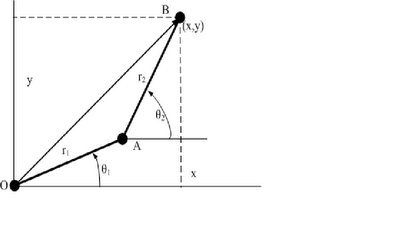## 10/25/2006

### 6.2 自訂函數之型式

function [輸出變數]=Name_of_function(輸入參數)

`function y = freebody(time)% calculation of height with respect to time% time: the elapsed time in seconds%    y:the depth of falling, cm% Example:  yy=freebody(1:20)y =1/2*980*time.*time;％y=(1/2)gt^2`

`>> yy=freebody(0:4:20)  yy =0        7840       31360       70560      125440      196000`

`>> help freebody time: the elapsed time in seconds    y:the depth of falling, cm Example:  yy=freebody(1:20)`

`　　　y =1/2*980*time.*time; ％y=(1/2)gt^2``function [x,y] = two_vectors(r1,r2,theta1,theta2)% Find the resultant position of two vectors% r1,r2: lengths of the two vectors, cm% theta1, theat2: horizontal angles of r1 & r2, deg.%  x,y:coordinates of the tip position.cm% Example:  [xx,yy]=two_vectors(5,8,30,60)d2r=pi/180;th1=theta1*d2r;th2=theta2*d2r;x =r1.*cos(th1)+r2.*cos(th2);y =r1.*sin(th1)+r2.*sin(th2);`

`>> [xx,yy]=two_vectors(5,8,20,50)xx =    9.8408yy =    7.8385`

Ching 吳子青 提到...